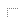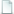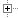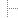1. The main features2. Controls4. Graphical Editor7. Table editor8. Database of materials9. Combinations11. Reinforced concrete structures calculation12. Report generating13. Calculator of the system14. Working with the demo version15. Examples

# 1. The main features

SELENA - a professional software complex intended to analyze the stress-strain state of a variety of constructions.

## Basic functionality:

 determine the deflected mode of construction from static actions and their combinations (concentrated forces, concentrated moments, displacements of supports, load distributed under the given law, the volumetric load, distributed moments, temperature expansion, drop of temperature, parametric loads); perform geometrically non-linear analysis of constructions taking into account longitudinal bending (P -Delta state); impose on the construction nonlinear and unilateral constraints; determine the buckling critical parameters of constructions with buckling length and slenderness of the beam members determination (linear analysis); determine the buckling critical parameters of the shells (non-linear analysis); program provides a unique test for buckling analysis of beam constructions, based on the theory of the small initial imperfections of the beam members; perform calculations of cable constructions and cable grid nets; form the programs of cables tension adjustment; determine the design cables tension of masts; determine the natural frequencies and modes of vibrations of the systems with localized masses (taking into account the longitudinal stress state and an automatic reduction systems with distributed masses to the systems with localized masses); determine the natural frequencies and (forms) modes of vibrations of the systems with distributed masses (taking into account the longitudinal stress); determine the local mass by the known natural frequencies (mass fitting); determine the deflected mode due to the harmonic effects nodal forces, nodal moments, displacements of supports with dynamic vibration absorbers taking into account longitudinal stress and individual energy absorption in structure members (the calculation is performed in the complex numbers that makes it possible to simulate "dry friction"); design construction to arbitrary and raised stationary random effects; perform calculations for arbitrary (changing over time) impacts (square and saw-tooth pulses, packets of pulses, the impact posed by an algebraic expression, simulation of stationary random effects, etc. (up to 20 types of disturbances)); to carry out the structure analysis due to the wind pulsation in the ground layer; perform tower type structures analysis due to the vortex excitation (wind resonance); perform calculations for the seismic effects; construct amplitude-frequency responses of the system due to the stationary and inertial dynamic effects; perform the optimal settings of dynamic absorbers of vibration; perform structure analysis due to the dynamic effects of the movable load; carry out construction and knurling the lanes; find the most unfavorable loading combinations; form arbitrary beam section with calculation of the geometric characteristics (including the moment of inertia of the torsion calculated by direct solution of Poisson problem for the area bounded circuit (circuits for multiply connected regions)) section; arbitrary shape thin-walled section with calculation of the provisions of Shear-center and sectorial moment of inertia; maintain a database of beam sections.

The complex oriented to the widespread use in the design, engineering and research organizations. It can also be used as the tutorial program in higher education.